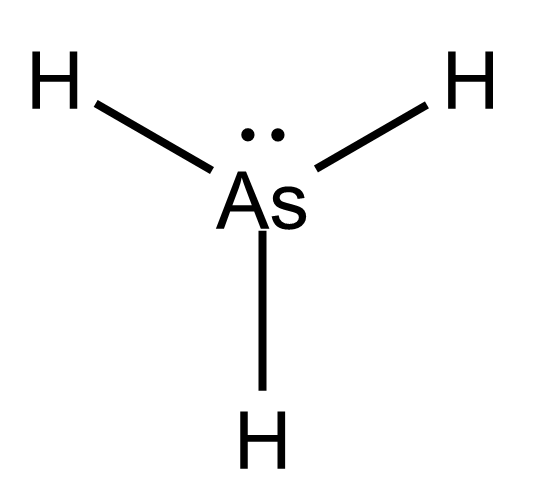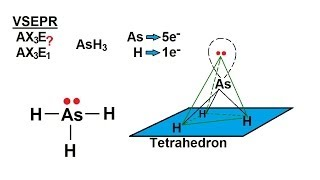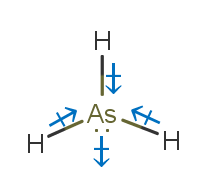# AsH3 Lewis Structure, Geometry, Hybridization, and PolarityArsine is an inorganic compound. It is the hydride of Arsenic metalloid. Arsenic is a member of group 15.
Arsine is a colorless gas with a garlic-like odor. It is flammable and highly toxic.

The gas is water-soluble and is transported in cylinders as liquefied compressed gas. AsH3 can be synthesized according to the following reaction.

4AsCl3   +   3NaBH4    —->   4 AsH3  +  3 NaCl  +  3BCl3

In this article, we will understand the bonding in AsH3. We will learn to draw Lewis Structures, predict shape, geometry, hybridization, and polarity for the given compound.

Contents

## AsH3 Lewis Structure

In the Lewis structure of atoms, we draw dots around the element’s chemical symbol.

The dots represent the valence electrons in that particular atom. It helps us get an idea about the type and number of bonds formed by the atom.

Lewis Structure of a compound is formed by arranging the atoms so that all the atoms obey the octet rule and formal charge is satisfied.

This 2-D arrangement of atoms gives us a rough estimate of the bonds formed between different atoms.

### Octet Rule

Atoms of all main group elements want to have a fully filled valence configuration like that of the noble gas.
Noble gases have eight valence electrons (except He), and all atoms form bonds to attain this configuration.

This preference for 8 electrons in the valence shell of main group elements gives rise to the octet rule.

### Formal Charge

It is a theoretical concept. If a compound is neutral, it does not mean that all atoms forming that compound are neutral. Sometimes, it is neutral due to the cancellation of charges.

The formal charge represents the charge present on each atom in a compound when the electrons are shared equally. Electronegativity is not considered. It is calculated using the formula

Formal charge= (valence electrons in isolate state of a neutral atom)- ( non-bonding valence electrons on atom)- ( number of bonds formed by atom)

### Steps to draw the lewis structure of AsH3

Step 1. Count the total number of valence shell electrons on the compound.

This is done by adding the valence shell electrons of all the constituent atoms.

 Atom Atomic Number Group Number Valence  electrons according to group number Electronic configuration (E.C.) Valence shell from E.C. Valence electrons from E.C. One As 33 15 5 1s2 2s2 2p6 3s2   3p6 4s2 3d10 4p3 n=4 5 Three H 1 1 1 1s1 n=1 1 Total number of valence shell electrons= 5 + (1*3) = 8

The Lewis dot structure for As and H are as follows-Step 2. Choose a suitable central atom for the compound.

The central atom is supposed to be the least electronegative one out of the constituent atoms. The central atom is supposed to share its electron density with all other atoms.

If the central atom is more electronegative than the side atom, the central atom will not share the electron density with side atoms.

Thus, As is the central atom for this compound.

Step 3. Draw a skeletal diagram.In this step, we have to arrange the side atoms and central atoms suitably.

Step 4. Arrange the valence electrons around the elemental symbols.The total valence shell electrons (calculated in step 1) are placed according to bond formation.

Step 5. Complete the octet of atoms by forming bonds.Each H has one valence electron in the isolated state. They share one electron with As to have a fully filled valence shell configuration.

As has five valence electrons in the isolated state. It shares one electron from all H-atoms to complete the octet.

Step 6. Calculate the formal charge on all atoms.

The net charge on this compound is zero. Therefore, the sum of formal charge on three atoms should come out to be zero.

 Atom Total number of  valence electrons in a free  atom Total number of  lone pairs (Total number of  bonding electrons)*0.5 Formal Charge As 5 1 6*0.5=3 4-0-4=0 H1 1 0 2*0.5=1 1-0-1=0 H2 1 0 2*0.5=1 1-0-1=0 H3 1 0 2*0.5=1 1-0-1=0

Thus, the structure drawn in step 5 is the best Lewis structure for AsH3.

## AsH3 GeometryLewis structure fails to predict an accurate geometry and shape of a compound. VSEPR theory helps in overcoming this drawback.

Geometry is the 3D arrangement of atoms in a molecule. It is essential from the academic point of view.

We do not need any theory for diatomic compounds as diatomic compounds are always linear. Different shapes arise for polyatomic compounds.

VSEPR theory stands for valence shell electron pair repulsion theory. Ronald Gillespie and Ronald Nyholm developed it.

VSEPR theory helps predict the geometry and shape of covalent compounds.

According to VSEPR theory-

The valence electron pairs repel each other, and this leads to instability.

• To make the arrangement of the electrons stable, the repulsions between them have to be decreased.

• As a result, electrons align themselves so that the repulsion is the least, and the distance between them is maximum.

• The stable arrangement of the valence electron pairs of atoms helps determine the molecular geometry.

Valence shell electrons involved in bonding are known as bonding pairs of electrons (bp), and those valence shell electrons that are not involved in bonding are termed as lone pairs of electrons (lp).

The terms geometry and shape are often confused with each other. The term geometry describes the orientation of lone pair of electrons as well in the compound while shape excludes the lone pair. The shape includes the effect of lone pair on other bonds.

### How to Predict Geometry of AsH3 Using VSEPR

1. Count the number of valence shell electrons on the central atom and let it be equal to A (arbitrary variable).

In the case of AsH3, the central atom is As. As has 5 valence electrons. (Shown in step1 of drawing lewis structure)

A=5

2. Count the number of side atoms and let it be equal to B (arbitrary variable).

In AsH3, there are three side atoms (hydrogen) and B=3

3. If the compound is charged, subtract the charge from B for the positively charged compound and add the charge to B for the negatively charged compound. This step can be avoided for neutral compounds.

In AsH3, there is no contribution of charge and B=3 only.

4. Add the contribution of side atoms and charge to the contribution of the central atom, i.e., A+B

For AsH3, A+B=8

5. Divide A+B by 2 to find total electron pairs affecting the shape.

For AsH3, there are 4 electron pairs.

6. Divide the total electron pairs as bonding and non-bonding. The bonding electron pair is equal to the number of side atoms.

For AsH3, there are three side atoms. Thus, there are three bonding pairs of electrons and one non-bonding pair of electrons.

Using this information, we can predict geometry and shape using the following table. Electron geometry is tetrahedral, and shape is trigonal pyramidal.## AsH3 Hybridization

The geometry and bonding of some polyatomic covalent compounds are explained using a unique concept called hybridization.

Hybridization is the mixing of atomic orbitals to form equivalent hybrid orbitals. It involves the redistribution of energy.

All atomic orbitals cannot be mixed. Only those orbitals that are similar in shape, size, and energy can mix and form hybrid orbitals.For example, one 3s and one 3p orbital can be mixed to form two sp orbitals, but 1s and 5d cannot because the energy difference is very high.

### Drago’s Rule

According to Drago’s rule, there is no need for hybridization for explaining bonding in some polyatomic compounds.

Those compounds have to fulfill the following criteria-

• The central atom should have at least one lone pair.

• The central atom should be from period 3 to 7.

• The electronegativity of the surrounding atom is less than ~2.5

When these conditions are followed, the energy difference between atomic orbitals is very high, and hence, they do not undergo mixing to form hybrid orbitals.

In AsH3, the central atom is unhybridized because-

AsH3 has one lone pair on the central atom.

• The central atom belongs to period 4.

• Side atoms have electronegativity=2.2.

In AsH3, pure p orbitals of As overlap with s orbitals of H to form a bond.

AsH3 does not undergo hybridization, but

• AsF3 does because side atoms are highly electronegative.

• NH3 does because the central atom belongs to period 2. Check out the article NH3 lewis structure.

• SiH4 does because the central atom does not have lone pair. Check out the article on SiH4 lewis structure.

## AsH3 PolarityThe polarity of a compound depends on the presence or absence of net dipole moment. The net dipole moment, in turn, depends on-

Dipole moments of individual bonds

• The difference in electronegativities of the constituent atoms

• Geometry/Symmetry of compound

In AsH3, there is only one type of bond, the As-H bond. The electronegativity of As and H is 2.18 and 2.20, respectively according to the first scale of electronegativity developed by Linus Pauling.

The electronegativity difference comes out to be 0.02, and hence the bond is polar and covalent.

A polar bond does not guarantee a polar compound.

Dipole moment is a vector quantity. Dipole moment vectors of bonds can get canceled if the shape of the compound is symmetrical.

In AsH3, along with 3 polar bonds, one lone pair is present, which distorts the geometry. The dipole moment vectors do not cancel out in the resultant trigonal pyramidal shape.

Hence, the AsH3 is polar.

## Conclusion

AsH3 is an inorganic compound.

The lewis structure obtained obeys the octet rule and satisfies the formal charge, and hence it is the most stable Lewis structure.

Arsine has tetrahedral geometry and trigonal pyramidal shape.

It is an unhybridized compound because the energy difference between atomic orbitals is high and they cannot form hybrid orbitals.

It is a polar compound because of the difference in electronegativity between As and H.